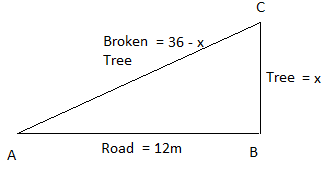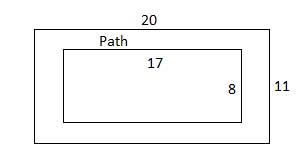# TCS NQT Ninja and Digital aptitude questions - 28

### 1. 11, 23, 47, 83, 131, . What is the next number?

a. 145
b. 178
c. 176
d. 191
Explanation:
11,23,47,83,131
23–11 = 12
47–23 = 24
83–47 = 36
131–83 = 48
Therefore, 131+60=191

### 2. A series of book was published at seven year intervals.  When the seventh book was published the total sum of publication year was 13, 524.  First book was published in?

a. 1911
b. 1910
c. 2002
d. 1932
Explanation:
Let the years be n, n+7, n+14, ...., n+42.  ($\because$ use formula ${T_n} = a + (n - 1)d$ to find nth term)
Sum = ${S_n} = \dfrac{n}{2}\left( {2a + (n - 1)d} \right)$ = $\dfrac{7}{2}\left( {2n + (7 - 1)7} \right)$ = 13,524
$\Rightarrow 7n + 147 = 13,524$
$\Rightarrow$ n = 1911

### 3. Crusoe hatched from a mysterious egg discovered by Angus, was growing at a fast pace that Angus had to move it from home to the lake. Given the weights of Crusoe in its first weeks of birth as 5, 15, 30,135, 405, 1215, 3645. Find the odd weight out.

a) 3645
b) 135
c) 30
d) 15
Explanation:
5×3 = 15
15×3 = 45 $\Rightarrow$ Given as 30
45×3 = 135
135×3 = 405
405×3 = 1215
1215×3 = 3645

### 4. A can complete a piece of work in 8 hours, B can complete in 10 hours and C in 12 hours. If A,B, C start the work together but A laves after 2 hours. Find the time taken by B and C to complete the remaining work.

1) 2 (1/11) hours
2) 4 (1/11) hours
3) 2 (6/11) hours
4) 2 hours
Explanation:
A,B,C's 1 hour work is = $\dfrac{1}{8} + \dfrac{1}{{10}} + \dfrac{1}{{12}}$ =  $\dfrac{{15 + 12 + 10}}{{120}} = \dfrac{{37}}{{120}}$
A,B,C worked together for 2 hours, Therefore, 2 hours work is = $\dfrac{{37}}{{120}} \times 2 = \dfrac{{37}}{{60}}$
Remaining work = $1 - \dfrac{{37}}{{60}} = \dfrac{{23}}{{60}}$
(23/60 work is done by B and C together)
B, C's 1 hour work = $\dfrac{1}{{10}} + \dfrac{1}{{12}} = \dfrac{{6 + 5}}{{60}} = \dfrac{{11}}{{60}}$
${\left( {\dfrac{{23}}{{60}}} \right)^{th}}$ part of the work done by B, C in = $\dfrac{{\left( {\dfrac{{23}}{{60}}} \right)}}{{\dfrac{{11}}{{60}}}}$ = $2\dfrac{1}{{11}}$ hours.

### 5. A tree of height 36m is on one edge of a road broke at a certain height.  It fell in such a way that the top of the tree touches the other edge of the road. If the breadth of the road is 12m, then what is the height at which the tree broke?

a. 16
b. 24
c. 12
d. 18
Explanation:
Let the tree was broken at x meters height from the ground and 36 - x be the length of other part of the tree.From the diagram, ${\left( {36 - x} \right)^2} = {x^2} + {12^2}$
$\Rightarrow 1296 - 72x + {x^2} = {x^2} + 144$
$\Rightarrow 72x = 1296 - 144$
$\Rightarrow x = 16$

### 6. The sticks of same length are used to form a triangle as shown below.If 87 such sticks are used then how many triangles can be formed?Explanation:
First triangle is formed by using 3 sticks, but any subsequent triangle may be formed by using 2 sticks.  Therefore, If 1st triangles uses 3 sticks, Remaining sticks = 87 - 3 = 84.  With these 84, we can form 42 triangles. So total = 42 + 1 = 43
Shortcut:
To solve questions like these, use formula, 2n + 1 = k.  Here n = triangles, k = sticks
2n+1 = 87 $\Rightarrow$ n = 43.

### 7. 17 × 8 m rectangular ground is surrounded by 1.5 m width path. Depth of the path is 12 cm. Gravel is filled and find the quantity of gravel required.

a. 5.5
b. 7.5
c. 6.05
d. 10.08
Explanation:Area of the rectangular ground = $17 \times 8 = 136{m^2}$
Area of the big rectangle considering the path width = $\left( {17 + 2 \times 1.5} \right) \times \left( {8 + 2 \times 1.5} \right) = 220{m^2}$
Area of the path = $220 - 136 = 84{m^2}$
Gravel required = $84{m^2} \times \dfrac{{12}}{{100}}m = 10.08{m^3}$

### 8. A sum of Rs.3000 is distributed among A, B, and C. A gets 2/3 of what B and C got together and c gets 1/3 of what A and B got together, C's share is?

Explanation:
Let B+C together got 3 units, then A get 2 units. or $\dfrac{{B + C}}{A} = \dfrac{3}{2}$ - - - (1)
Let A+B together got 3 units, then B get 1 units. or $\dfrac{{A + B}}{C} = \dfrac{3}{1}$ - - - (2)
By using Componendo and Dividendo, we can re-write equations (1) and (2), $\dfrac{{A + B + C}}{A} = \dfrac{{3 + 2}}{2} = \dfrac{5}{2} = \dfrac{{20}}{8}$ and $\dfrac{{A + B + C}}{C} = \dfrac{{3 + 1}}{1} = \dfrac{4}{1} = \dfrac{{20}}{5}$
So A = 8, B = 7, C = 5
C's share = $\dfrac{5}{{(8 + 5 + 7)}} \times 3000 = 750$

### 9. The numbers 272738 and 232342, when divided by n, a two digit number, leave a remainder of 13 and 17 respectively. Find the sum of the digits of n?

a. 7
b. 8
c. 5
d. 4
Explanation:
From the given information, (272738 - 13, 232342 - 17) are exactly divisible by that two digit number.
We have to find the HCF of the given numbers 272725, 232325.
HCF = 25.
So sum of the digits = 7.

### 10.  Assume that f(1)=0 and f(m+n)=f(m)+f(n)+4(9mn-1).  For all natural numbers (Integers>0)m and n.  What is the value of f(17)?

a. 5436
b. 4831
c. 5508
d. 4832
Explanation:
f(1) = 0
f(2) = f(1+1) = f(1)+f(1)+4(9×1×1 – 1) = 0+0+4×8 = 32
f(4) = f(2+2) = f(2)+f(2)+4(9×2×2 – 1) = 32+32+4×35 = 204
f(8) = f(4+4) = f(4)+f(4)+4(9×4×4 – 1) = 204+204+4×143 = 980
f(16) = f(8+8) = f(8)+f(8)+4(9×8×8 – 1) = 980+980+4×575 = 4260
f(17) = f(1+16) = f(16)+f(1)+4(9×16×1 –1) = 4260+0+ 4×143 = 4832